# How to use Equation of Motion

Continuing from the previous lecture, we will talk about the second law of motion.
Last time we focused on the content of the law, but this time we will focus on calculations.

“The acceleration produced in an object is proportional to the magnitude of the force acted and inversely proportional to its mass.”

In the last lecture we expressed this with the equation F=ma.
What can this equation be used for?

## How to use the law of motion

F=ma. Look closely at this equation.

Since it is an equation, we can find the unknown value by substituting a numerical value for the letter.
For example, if we know the values of mass m and acceleration a, we can find the force F by substituting them into this equation!

Think back to what you have learned so far.

The force F acting on an object can be calculated separately since we have already learned how to calculate it: F=mg for gravitational force, F=kx for elastic force, and so on.

This means that the equation F=ma in the second law of motion can be used to find a by substituting F and m!

If we write F=ma, it will look like “an equation to find F“, so from now on we will write this expression as “ma=F“.
Although the left and right sides are simply interchanged, the nuance of F is not something to be sought, but something to be substituted” is shown.

This equation is called the “Equation of motion” and is very important when studying physics!

## Equation of motion tells us the past or future of an object

Before we begin specific calculations, let’s first understand the significance of equation of motion!

If the force F acting on the object is constant, the object will have a uniform accelerated motion (Equation of motion can be used even if F is not constant, but we prefer simplicity).

If it is a uniform accelerated motion, we can use the three formulas we did before to find “the velocity after t seconds from the start” and “the displacement after t seconds”.

However, to use the equation for uniform accelerated motion, the acceleration a must be substituted.
It’s hard to find the acceleration directly, but the equation of motion is easy to calculate!

The equation of motion requires the mass m and the force F acting on the object.

To summarize the above, If we know the mass m and the force F acting on the object, we can calculate the velocity and position of the object after t seconds!

This is amazing!
Solving the equation of motion tells us what motion the object has been in and what motion it will be in the future!

Most importantly, the equation of motion holds for any object.
Not only free-falling objects and pendulums, but also, for example, the motion of stars can be calculated with the equation of motion.

The date of the next total solar eclipse can be identified because the motion of the sun and moon is calculated using equations of motion. How exciting to learn about equations of motion that allow us to do calculations like these!

## How to formulate the equation of motion

Let’s take a concrete problem as an example to formulate the equation of motion.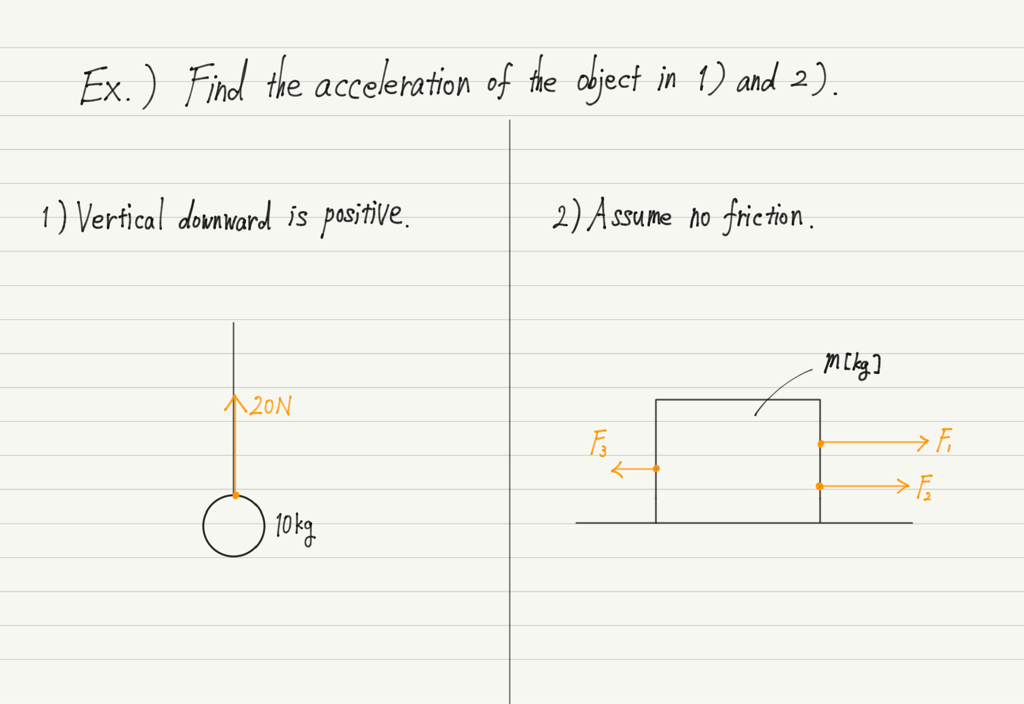When you are asked to find the acceleration in a problem involving forces, first think of the equation of motion.

The important thing with equations of motion is to formulate the equation rather than to calculate it.
Here are some steps on how to formulate an equation of motion!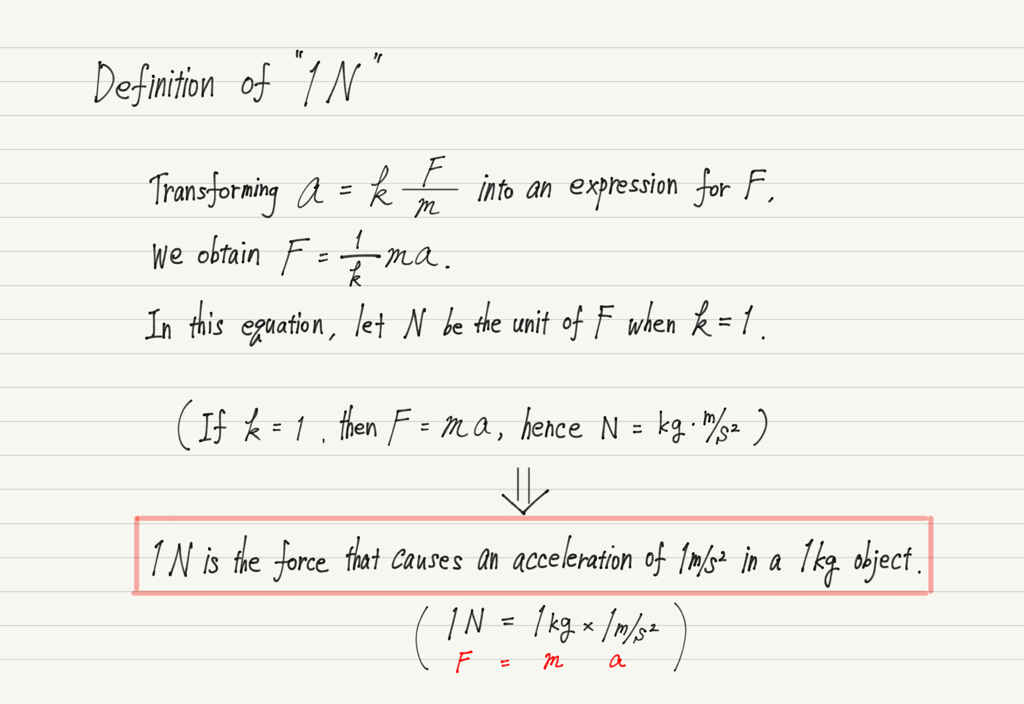Let’s try it out with the previous example. First, write down the forces acting on the object.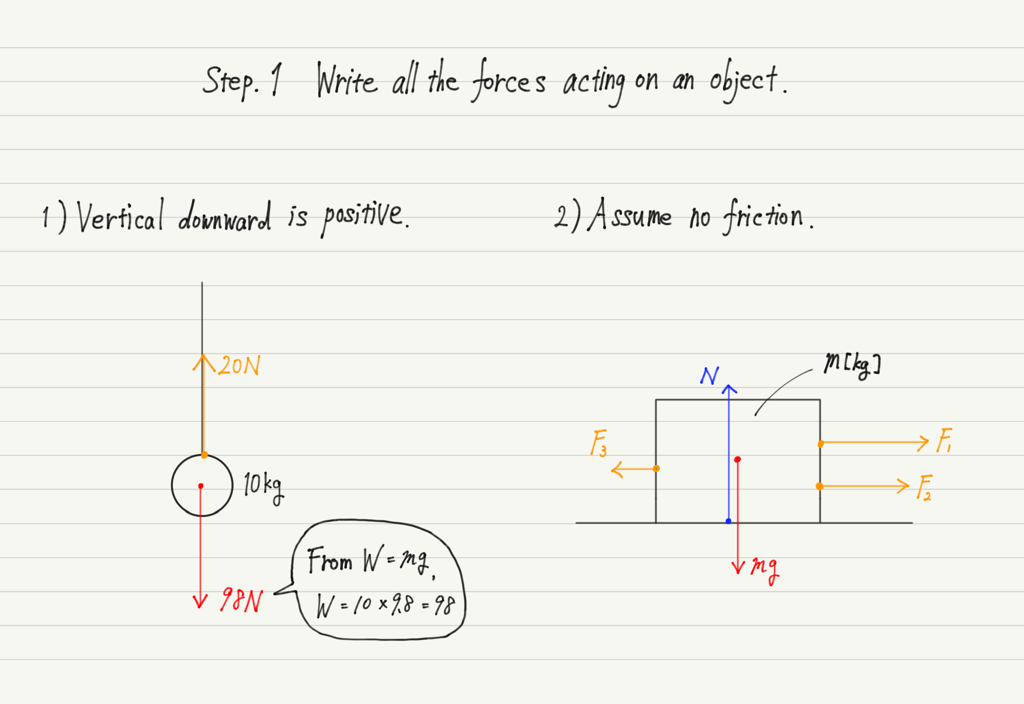Now that we have written all the forces acting on the object, we next check the direction of the acceleration a.

In 1), the problem statement says the direction is vertical downward, but in 2), nothing is written.
If nothing is written, assume the direction of acceleration by yourself.
This time, let’s make the right orientation positive.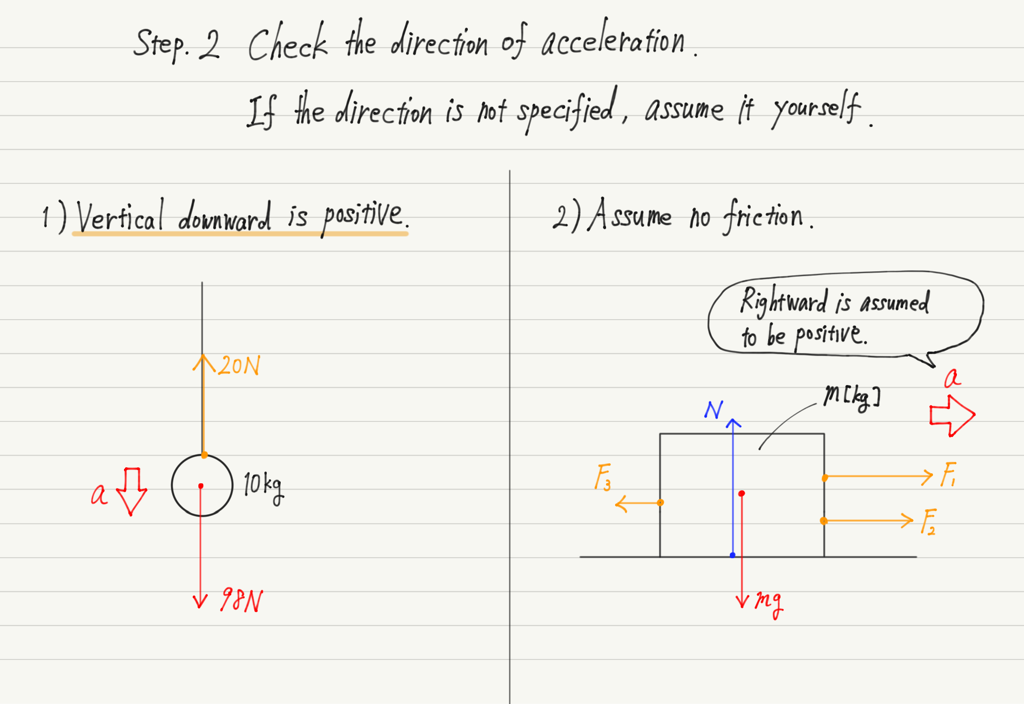Now we are ready!　Finally, it is time to formulate step 3, the equation of motion.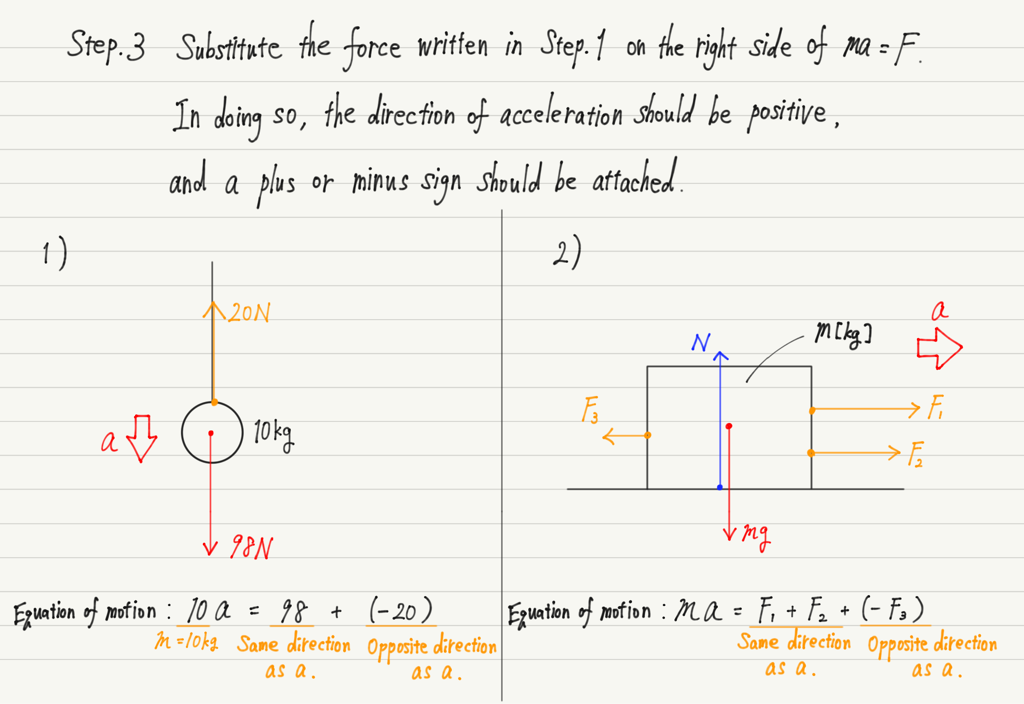The form of the equation of motion is ma=F, but the left-hand side, ma, remains the same for any object in any motion.
Therefore, whenever you are asked to “formulate an equation of motion,” you may begin by writing “ma =”.

1) is given a mass of 10 kg, so let us substitute 10 for m.

The important part is the right-hand side, where F is the composed force acting on the object, so we will sum up the forces we have just written down.
But, since force has a direction, it cannot just be added. Remember to add a minus sign to the force in the opposite direction, based on the direction of acceleration you checked in step 2.

Note that mg and N are not included in the equation of motion in this example.
The reason is that in 2), the object moves either to the right or to the left.
No matter how much upward or downward force is acted, it has nothing to do with the left or right motion.

Therefore, the right hand side of the equation of motion does not include the force perpendicular to the acceleration!

However, in step 1, be sure to write all forces acting on the object, regardless of whether they are used or not. This is because even if they are not used in the equation of motion, they may be used in other calculations.

When you’re done here, your equation of motion is complete!
Once you have the equation of motion, all you have to do is solve it to find a.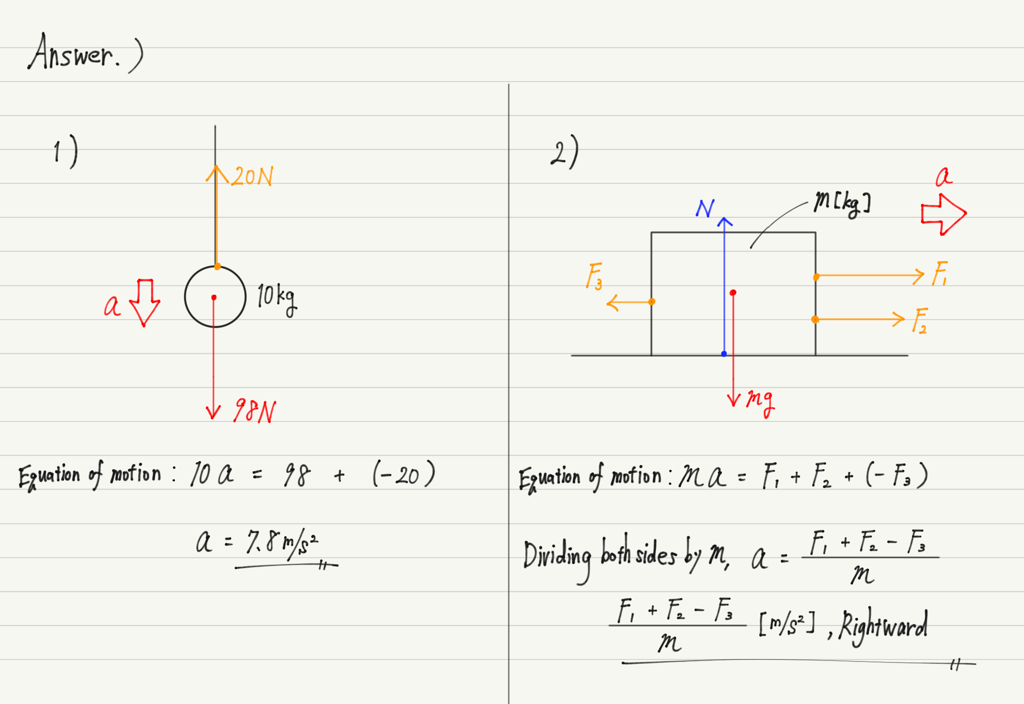“Formulating the equation of motion” sounds difficult, but all you actually have to do is write down the forces, add a sign, and add them together.

## Summary of this lecture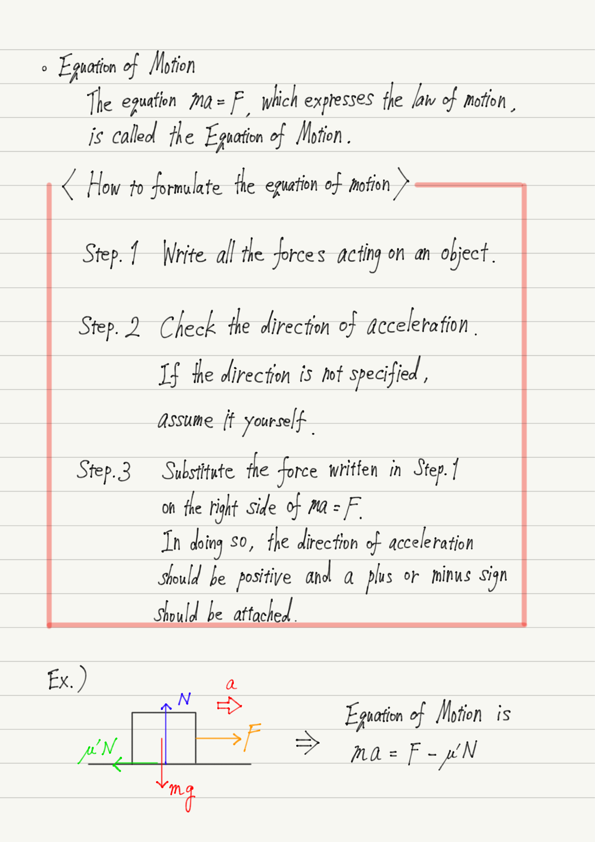## Next Time

We will begin the topic of energy in the next lecture.

https://www.yukimura-physics.com/entry/dyn-f23

error: Content is protected !!